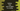# Python program to find the sum of digits in a string### Python program to find the sum of digits in a string:

This tutorial will show you how to find the sum of digits in a string. We will learn different ways to solve this problem. The program will read one string as user input and it will calculate the sum of all digits in that string.

To solve this problem, we will iterate through the characters of the string one by one. Using isdigit() method, we can check if one character is digit or not. If it is a digit, we will add its value to a different sum variable that holds the total sum of all digits.

### Using a loop :

Let’s try to do this by using a for loop. This loop will iterate through the characters of the string one by one and if it find any digit, it will add it to the final sum.

Let’s take a look at the below program:

``````def sum_digits(str):
sum = 0
for c in str:
if c.isdigit() == True:
sum += int(c)

return sum

given_str = input("Enter a string : ")
print(sum_digits(given_str))``````

In this program, we have defined one function sum_digits to find the sum of all digits of a string. It takes one string as parameter and returns the sum of all numbers found in the string.

We are using one for loop to find if a character is digit or not using isdigit() method. If it returns True, we are adding the integer value of that character to sum.

### Sample output:

``````Enter a string : hello123
6

Enter a string : hello123 world45
15``````

### Writing it in one line:

We can also write the same program in one line.

``````def sum_digits(str):
return sum(int(c) for c in str if c.isdigit())

given_str = input("Enter a string : ")
print(sum_digits(given_str))``````

We are doing the same thing in this program but in one line. If you run this program, it will print the same output.